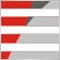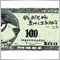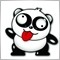# 求移植一个指标算法，价格合理的联系12

• 这几天在网上查资料关于STOCHRSI这个指标，都描述的看不太懂。

```/*
LC := REF(CLOSE,1); //REF(C,1) 上一周期的收盘价
RSI:=SMA(MAX(CLOSE-LC,0),N,1)/SMA(ABS(CLOSE-LC),N,1) *100;
％K:     MA(RSI-LLV(RSI,M),P1)/MA(HHV(RSI,M)-LLV(RSI,M),P1)*100;  LLV（l,60）表示：检索60天内的最低价，可适应于检索任何股票
％D:MA(％K,P2);

LC := REF(CLOSE,1);
RSI:=SMA(MAX(CLOSE-LC,0),N,1)/SMA(ABS(CLOSE-LC),N,1) *100;
STOCHRSI:MA(RSI-LLV(RSI,M),P1)/MA(HHV(RSI,M)-LLV(RSI,M),P1)*100;
*/
```40

littlelittleD:
• 这几天在网上查资料关于STOCHRSI这个指标，都描述的看不太懂。1492847260